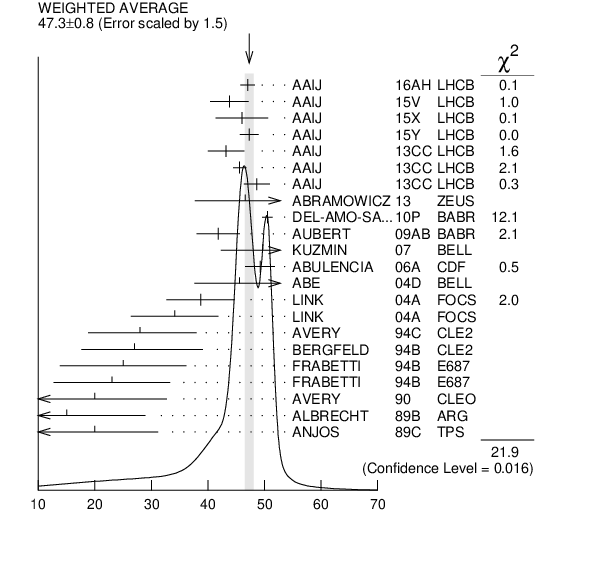${{\boldsymbol D}_{{2}}^{*}{(2460)}}$ WIDTH

VALUE (MeV) EVTS DOCUMENT ID TECN CHG  COMMENT
 $\bf{ 47.3 \pm0.8}$ OUR AVERAGE  Error includes scale factor of 1.5. See the ideogram below.
$47.0$ $\pm0.8$ $\pm1.0$ 28k 1
 2016 AH
LHCB 0 ${{\mathit B}^{-}}$ $\rightarrow$ ${{\mathit D}^{+}}{{\mathit \pi}^{-}}{{\mathit \pi}^{-}}$
$43.8$ $\pm2.9$ $\pm1.8$ 2k 2
 2015 V
LHCB 0 ${{\mathit B}^{-}}$ $\rightarrow$ ${{\mathit D}^{+}}{{\mathit K}^{-}}{{\mathit \pi}^{-}}$
$46.0$ $\pm3.4$ $\pm3.2$ 3
 2015 X
LHCB + ${{\mathit B}^{0}}$ $\rightarrow$ ${{\overline{\mathit D}}^{0}}{{\mathit K}^{+}}{{\mathit \pi}^{-}}$
$47.3$ $\pm1.5$ $\pm0.7$ 4
 2015 Y
LHCB + ${{\mathit B}^{0}}$ $\rightarrow$ ${{\overline{\mathit D}}^{0}}{{\mathit \pi}^{+}}{{\mathit \pi}^{-}}$
$43.2$ $\pm1.2$ $\pm3.0$ 82k
 2013 CC
LHCB 0 ${{\mathit p}}$ ${{\mathit p}}$ $\rightarrow$ ${{\mathit D}^{*+}}{{\mathit \pi}^{-}}{{\mathit X}}$
$45.6$ $\pm0.4$ $\pm1.1$ 675k
 2013 CC
LHCB 0 ${{\mathit p}}$ ${{\mathit p}}$ $\rightarrow$ ${{\mathit D}^{+}}{{\mathit \pi}^{-}}{{\mathit X}}$
$48.6$ $\pm1.3$ $\pm1.9$ 342k
 2013 CC
LHCB + ${{\mathit p}}$ ${{\mathit p}}$ $\rightarrow$ ${{\mathit D}^{0}}{{\mathit \pi}^{+}}{{\mathit X}}$
$46.6$ $\pm8.1$ ${}^{+5.9}_{-3.8}$ 2.3k 5
 2013
ZEUS 0 ${{\mathit e}^{\pm}}$ ${{\mathit p}}$ $\rightarrow$ ${{\mathit D}^{(*)+}}{{\mathit \pi}^{-}}{{\mathit X}}$
$50.5$ $\pm0.6$ $\pm0.7$ 243k
 2010 P
BABR 0 ${{\mathit e}^{+}}$ ${{\mathit e}^{-}}$ $\rightarrow$ ${{\mathit D}^{+}}{{\mathit \pi}^{-}}{{\mathit X}}$
$41.8$ $\pm2.5$ $\pm2.9$ 3.4k
 2009 AB
BABR 0 ${{\mathit B}^{-}}$ $\rightarrow$ ${{\mathit D}^{+}}{{\mathit \pi}^{-}}{{\mathit \pi}^{-}}$
$49.7$ $\pm3.8$ $\pm6.4$ 2909
 2007
BELL + ${{\mathit e}^{+}}$ ${{\mathit e}^{-}}$ $\rightarrow$ hadrons
$49.2$ $\pm2.3$ $\pm1.3$ 20k
 2006 A
CDF 0 1900 ${{\mathit p}}$ ${{\overline{\mathit p}}}$ $\rightarrow$ ${{\mathit D}^{+}}{{\mathit \pi}^{-}}{{\mathit X}}$
$45.6$ $\pm4.4$ $\pm6.7$ 6
 2004 D
BELL 0 ${{\mathit B}^{-}}$ $\rightarrow$ ${{\mathit D}^{+}}{{\mathit \pi}^{-}}{{\mathit \pi}^{-}}$
$38.7$ $\pm5.3$ $\pm2.9$ 5.8k 6
 2004 A
FOCS 0 ${{\mathit \gamma}}$ A
$34.1$ $\pm6.5$ $\pm4.2$ 3.5k 7
 2004 A
FOCS + ${{\mathit \gamma}}$ A
$28$ ${}^{+8}_{-7}$ $\pm6$ 486
 1994 C
CLE2 0 ${{\mathit e}^{+}}$ ${{\mathit e}^{-}}$ $\rightarrow$ ${{\mathit D}^{+}}{{\mathit \pi}^{-}}$ X
$27$ ${}^{+11}_{-8}$ $\pm5$ 310
 1994 B
CLE2 + ${{\mathit e}^{+}}$ ${{\mathit e}^{-}}$ $\rightarrow$ ${{\mathit D}^{0}}{{\mathit \pi}^{+}}$ X
$25$ $\pm10$ $\pm5$ 128
 1994 B
E687 0 ${{\mathit \gamma}}$ ${}^{}\mathrm {Be}$ $\rightarrow$ ${{\mathit D}^{+}}{{\mathit \pi}^{-}}$ X
$23$ $\pm9$ $\pm5$ 185
 1994 B
E687 + ${{\mathit \gamma}}$ ${}^{}\mathrm {Be}$ $\rightarrow$ ${{\mathit D}^{0}}{{\mathit \pi}^{+}}$ X
$20$ ${}^{+9}_{-12}$ ${}^{+9}_{-10}$ 440
 1990
CLEO 0 ${{\mathit e}^{+}}$ ${{\mathit e}^{-}}$ $\rightarrow$ ${{\mathit D}^{*+}}{{\mathit \pi}^{-}}$ X
$15$ ${}^{+13}_{-10}$ ${}^{+5}_{-10}$ 337
 1989 B
ARG 0 ${{\mathit e}^{+}}$ ${{\mathit e}^{-}}$ $\rightarrow$ ${{\mathit D}^{+}}{{\mathit \pi}^{-}}$ X
$20$ $\pm10$ $\pm5$ 153
 1989 C
TPS 0 ${{\mathit \gamma}}$ ${{\mathit N}}$ $\rightarrow$ ${{\mathit D}^{+}}{{\mathit \pi}^{-}}$ X
• • We do not use the following data for averages, fits, limits, etc. • •
$46.0$ $\pm1.4$ $\pm1.8$ 8
 2015 Y
LHCB + ${{\mathit B}^{0}}$ $\rightarrow$ ${{\overline{\mathit D}}^{0}}{{\mathit \pi}^{+}}{{\mathit \pi}^{-}}$
 1 From the amplitude analysis in the model describing the ${{\mathit D}^{+}}{{\mathit \pi}^{-}}$ wave together with virtual contributions from the ${{\mathit D}^{*}{(2007)}^{0}}$ and ${{\mathit B}^{*0}}$ states, and components corresponding to the ${{\mathit D}_{{2}}^{*}{(2460)}^{0}}$, ${{\mathit D}_{{1}}^{*}{(2680)}^{0}}$, ${{\mathit D}_{{3}}^{*}{(2760)}^{0}}$, and ${{\mathit D}_{{2}}^{*}{(3000)}^{0}}$ resonances.
 2 From the amplitude analysis in the model describing the ${{\mathit D}^{+}}{{\mathit \pi}^{-}}$ wave together with virtual contributions from the ${{\mathit D}^{*}{(2007)}^{0}}$ and ${{\mathit B}^{*0}}$ states, nonresonant spin-0 and spin-1 components as well as the ${{\mathit D}_{{0}}^{*}{(2400)}^{0}}$, ${{\mathit D}_{{2}}^{*}{(2460)}^{0}}$ and ${{\mathit D}_{{1}}^{*}{(2760)}^{0}}$ resonances.
 3 From the Dalitz plot analysis including various ${{\mathit K}^{*}}$ and ${{\mathit D}^{**}}$ mesons as well as broad structures in the ${{\mathit K}}{{\mathit \pi}}$ ${\mathit S}{\mathrm -wave}$ and the ${{\mathit D}}{{\mathit \pi}}$ $\mathit S$- and ${\mathit P}{\mathrm -wave}$s.
 4 Modeling the ${{\mathit \pi}^{+}}{{\mathit \pi}^{-}}$ ${\mathit S}{\mathrm -wave}$ with the Isobar formalism.
 5 From the combined fit of the ${{\mathit M}}$( ${{\mathit D}^{+}}{{\mathit \pi}^{-}}$ ) and ${{\mathit M}}$( ${{\mathit D}^{*+}}{{\mathit \pi}^{-}}$ ) distributions. and A$_{{{\mathit D}_{{2}}}}$ fixed to the theoretical prediction of $-1$.
 6 Fit includes the contribution from ${{\mathit D}_{{0}}^{*}{(2400)}^{0}}$.
 7 Fit includes the contribution from ${{\mathit D}_{{0}}^{*}{(2400)}^{\pm}}$.
 8 Modeling the ${{\mathit \pi}^{+}}{{\mathit \pi}^{-}}$ ${\mathit S}{\mathrm -wave}$ with the K-matrix formalism.${{\mathit D}_{{2}}^{*}{(2460)}}$ width (MeV)
References:
 AAIJ 2016AH
PR D94 072001 Amplitude analysis of ${{\mathit B}^{-}}$ $\rightarrow$ ${{\mathit D}^{+}}{{\mathit \pi}^{-}}{{\mathit \pi}^{-}}$ Decays
 AAIJ 2015Y
PR D92 032002 Dalitz Plot Analysis of ${{\mathit B}^{0}}$ $\rightarrow$ ${{\overline{\mathit D}}^{0}}{{\mathit \pi}^{+}}{{\mathit \pi}^{-}}$ Decays
 AAIJ 2015X
PR D92 012012 Amplitude Analysis of ${{\mathit B}^{0}}$ $\rightarrow$ ${{\overline{\mathit D}}^{0}}{{\mathit K}^{+}}{{\mathit \pi}^{-}}$ Decays
 AAIJ 2015V
PR D91 092002 First Observation and Amplitude Analysis of the ${{\mathit B}^{-}}$ $\rightarrow$ ${{\mathit D}^{+}}{{\mathit K}^{-}}{{\mathit \pi}^{-}}$ Decay
 AAIJ 2013CC
JHEP 1309 145 Study of ${{\mathit D}_{{J}}}$ Meson Decays to ${{\mathit D}^{+}}{{\mathit \pi}^{-}}$, ${{\mathit D}^{0}}{{\mathit \pi}^{+}}$ and ${{\mathit D}^{*+}}{{\mathit \pi}^{-}}$ Final States in ${{\mathit p}}{{\mathit p}}$ Collisions
 ABRAMOWICZ 2013
NP B866 229 Production of the Excited Charm Mesons ${{\mathit D}_{{1}}}$ and ${{\mathit D}_{{2}}^{*}}$ at HERA
 DEL-AMO-SANCHEZ 2010P
PR D82 111101 Observation of New Resonances Decaying to ${{\mathit D}}{{\mathit \pi}}$ and ${{\mathit D}^{*}}{{\mathit \pi}}$ in Inclusive ${{\mathit e}^{+}}{{\mathit e}^{-}}$ Collisions near $\sqrt {s }$ = 10.58 GeV
 AUBERT 2009AB
PR D79 112004 Dalitz Plot Analysis of
 KUZMIN 2007
PR D76 012006 Study of ${{\overline{\mathit B}}^{0}}$ $\rightarrow$ ${{\mathit D}^{0}}{{\mathit \pi}^{+}}{{\mathit \pi}^{-}}$ Decays
 ABULENCIA 2006A
PR D73 051104 Measurement of Mass and Width of the Excited Charmed Meson States ${{\mathit D}_{{1}}^{0}}$ and ${{\mathit D}_{{2}}^{*0}}$ at CDF
 ABE 2004D
PR D69 112002 Study of ${{\mathit B}^{-}}$ $\rightarrow$ ${{\mathit D}^{**0}}{{\mathit \pi}^{-}}$ ( ${{\mathit D}^{**0}}$ $\rightarrow$ ${{\mathit D}}{}^{(*)+}$ ${{\mathit \pi}^{-}}$ ) Decays
PL B586 11 Measurement of Masses and Widths of Excited Charm Mesons ${{\mathit D}_{{2}}^{*}}$ and Evidence for Broad States
PL B331 236 Production and Decay of ${{\mathit D}_{{1}}{(2420)}^{0}}$ and ${{\mathit D}_{{2}}^{*}{(2460)}^{0}}$
PL B340 194 Observation of ${{\mathit D}_{{1}}{(2420)}^{+}}$ and ${{\mathit D}_{{2}}^{*}{(2460)}^{+}}$
PRL 72 324 Measurement of the Masses and Widths of $\mathit L$ = 1 Charm Mesons
PR D41 774 P-wave Charmed Mesons in ${{\mathit e}^{+}}{{\mathit e}^{-}}$ Annihilation
PL B221 422 Observation of ${{\mathit D}^{*}{(2459)}^{0}}$ in ${{\mathit e}^{+}}{{\mathit e}^{-}}$ Annihilation